# Resources tagged with: Polyhedra

Filter by: Content type:
Age range:
Challenge level:

### There are 26 results

Broad Topics > 3D Geometry, Shape and Space > Polyhedra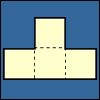### Cut Nets

##### Age 7 to 11Challenge Level

Each of the nets of nine solid shapes has been cut into two pieces. Can you see which pieces go together?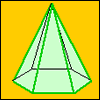### A Chain of Eight Polyhedra

##### Age 7 to 11Challenge Level

Can you arrange the shapes in a chain so that each one shares a face (or faces) that are the same shape as the one that follows it?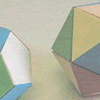### Face Painting

##### Age 7 to 11Challenge Level

You want to make each of the 5 Platonic solids and colour the faces so that, in every case, no two faces which meet along an edge have the same colour.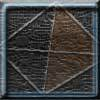### Tetrahedron Faces

##### Age 7 to 11Challenge Level

One face of a regular tetrahedron is painted blue and each of the remaining faces are painted using one of the colours red, green or yellow. How many different possibilities are there?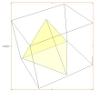### A Mean Tetrahedron

##### Age 11 to 14Challenge Level

Can you number the vertices, edges and faces of a tetrahedron so that the number on each edge is the mean of the numbers on the adjacent vertices and the mean of the numbers on the adjacent faces?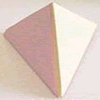### Child's Play

##### Age 7 to 11Challenge Level

A toy has a regular tetrahedron, a cube and a base with triangular and square hollows. If you fit a shape into the correct hollow a bell rings. How many times does the bell ring in a complete game?### Triangles to Tetrahedra

##### Age 11 to 14Challenge Level

Imagine you have an unlimited number of four types of triangle. How many different tetrahedra can you make?### Tetra Square

##### Age 11 to 14Challenge Level

ABCD is a regular tetrahedron and the points P, Q, R and S are the midpoints of the edges AB, BD, CD and CA. Prove that PQRS is a square.### Guess What?

##### Age 7 to 11Challenge Level

Can you find out which 3D shape your partner has chosen before they work out your shape?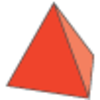### Next Size Up

##### Age 7 to 11Challenge Level

The challenge for you is to make a string of six (or more!) graded cubes.### Icosian Game

##### Age 11 to 14Challenge Level

This problem is about investigating whether it is possible to start at one vertex of a platonic solid and visit every other vertex once only returning to the vertex you started at.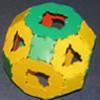### Platonic and Archimedean Solids

##### Age 7 to 16Challenge Level

In a recent workshop, students made these solids. Can you think of reasons why I might have grouped the solids in the way I have before taking the pictures?### Tetrahedra Tester

##### Age 11 to 14Challenge Level

An irregular tetrahedron is composed of four different triangles. Can such a tetrahedron be constructed where the side lengths are 4, 5, 6, 7, 8 and 9 units of length?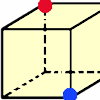### Redblue

##### Age 7 to 11Challenge Level

Investigate the number of paths you can take from one vertex to another in these 3D shapes. Is it possible to take an odd number and an even number of paths to the same vertex?### Thinking 3D

##### Age 7 to 14

How can we as teachers begin to introduce 3D ideas to young children? Where do they start? How can we lay the foundations for a later enthusiasm for working in three dimensions?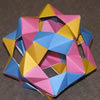### Modular Origami Polyhedra

##### Age 7 to 16Challenge Level

These models have appeared around the Centre for Mathematical Sciences. Perhaps you would like to try to make some similar models of your own.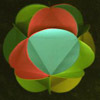### Making Maths: Triangular Ball

##### Age 7 to 11Challenge Level

Make a ball from triangles!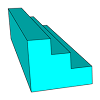### Which Solid?

##### Age 7 to 16Challenge Level

This task develops spatial reasoning skills. By framing and asking questions a member of the team has to find out what mathematical object they have chosen.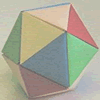### Paper Folding - Models of the Platonic Solids

##### Age 11 to 16

A description of how to make the five Platonic solids out of paper.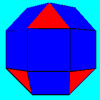### Rhombicubocts

##### Age 11 to 14Challenge Level

Each of these solids is made up with 3 squares and a triangle around each vertex. Each has a total of 18 square faces and 8 faces that are equilateral triangles. How many faces, edges and vertices. . . .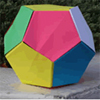### Dodecamagic

##### Age 7 to 11Challenge Level

Here you see the front and back views of a dodecahedron. Each vertex has been numbered so that the numbers around each pentagonal face add up to 65. Can you find all the missing numbers?### Classifying Solids Using Angle Deficiency

##### Age 11 to 16Challenge Level

Toni Beardon has chosen this article introducing a rich area for practical exploration and discovery in 3D geometry### Let's Get Flexible with Geometry

##### Age 5 to 11

In this article for primary teachers, Ems explores ways to develop mathematical flexibility through geometry.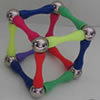### Magnetic Personality

##### Age 7 to 16Challenge Level

60 pieces and a challenge. What can you make and how many of the pieces can you use creating skeleton polyhedra?### Which Solids Can We Make?

##### Age 11 to 14Challenge Level

Interior angles can help us to work out which polygons will tessellate. Can we use similar ideas to predict which polygons combine to create semi-regular solids?### Lighting up Time

##### Age 7 to 14Challenge Level

A very mathematical light - what can you see?